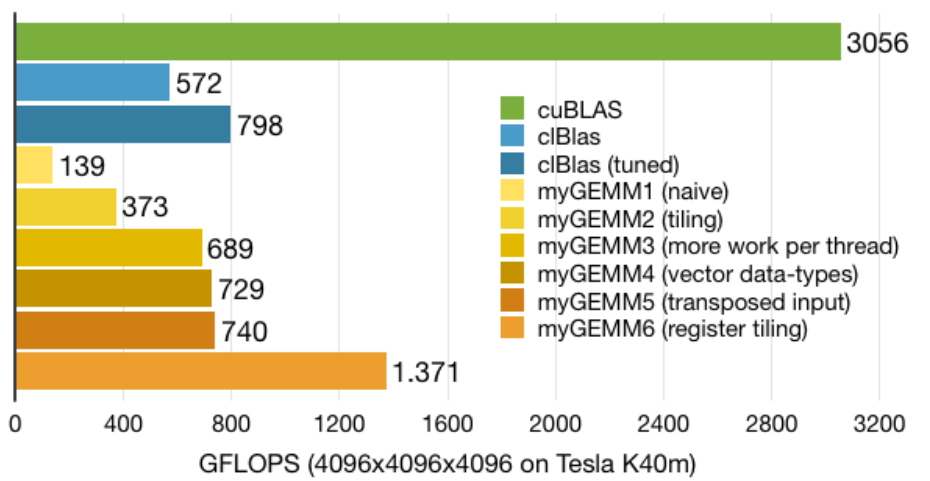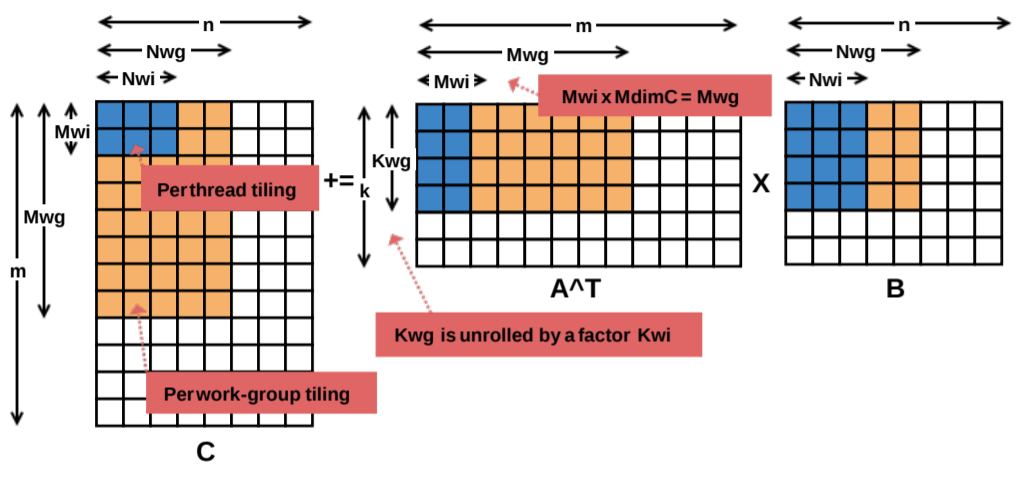# GEMM tests on GeForce RTX 2070

WebGL2-compute GEMM shaders based on Cedric Nugteren's tutorial (see his test results on Tesla below).GEMMs are tested on MSI GeForce RTX 2070 Aero + Intel i3-8100, Windows 10 64-bit, OpenGL backend, N = 4096.

"Semi-quantitative" plot. Unfortunately the shader 6 has long "warming up" (up to 50 iterations), e.g. for N = 2048
T(ms)= 33.7   33.8   33.2   32.3   34   20.4   5.4   5.2   5.2   5.2
Therefore it = 100 and "highest reproducible numbers" are used :)

For N = 1024 shader 6 is not optimized yet. As you can see below, tuned OpenCL kernel get 3.94 TFLOPS.

D3D11 backend has higher overheads for big N than OpenGL one.

## CLBlast GEMMs

Test resultes from CLBlast (N = 4096). There are compiled tuners for GEMM (for Linux and Windows) at CLBlast releases.
```* Found best result 20.25 ms: 6786.0 GFLOPS
* Best parameters: GEMMK=0 KREG=1 KWG=16 KWI=2 MDIMA=32 MDIMC=8 MWG=128
NDIMB=32 NDIMC=16 NWG=128 PRECISION=32 SA=1 SB=1 STRM=1 STRN=0 VWM=4 VWN=1
```
For N = 1024 tuner has found the same parameters and 3942.5 GFLOPS
```* Found best result 0.54 ms: 3942.5 GFLOPS
* Best parameters: GEMMK=0 KREG=1 KWG=16 KWI=2 MDIMA=32 MDIMC=8 MWG=128
NDIMB=32 NDIMC=16 NWG=128 PRECISION=32 SA=1 SB=1 STRM=1 STRN=0 VWM=4 VWN=1
```SGEMM in WebGL2-compute     updated 19 July 2019